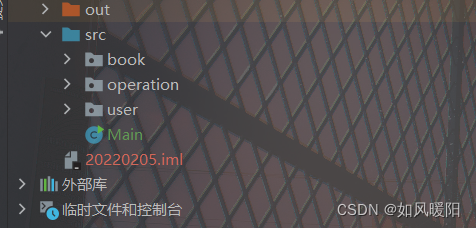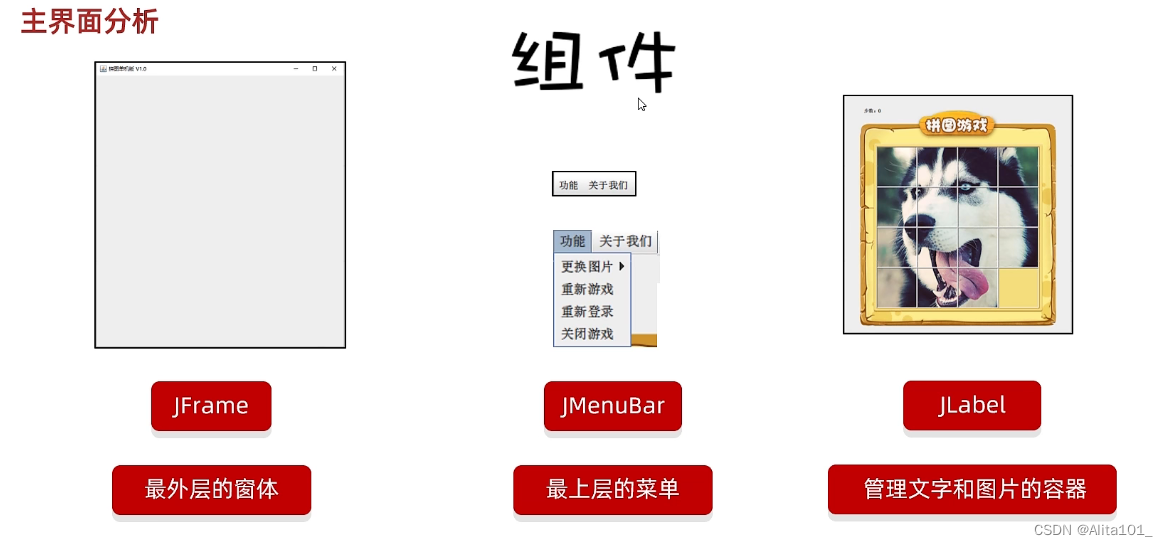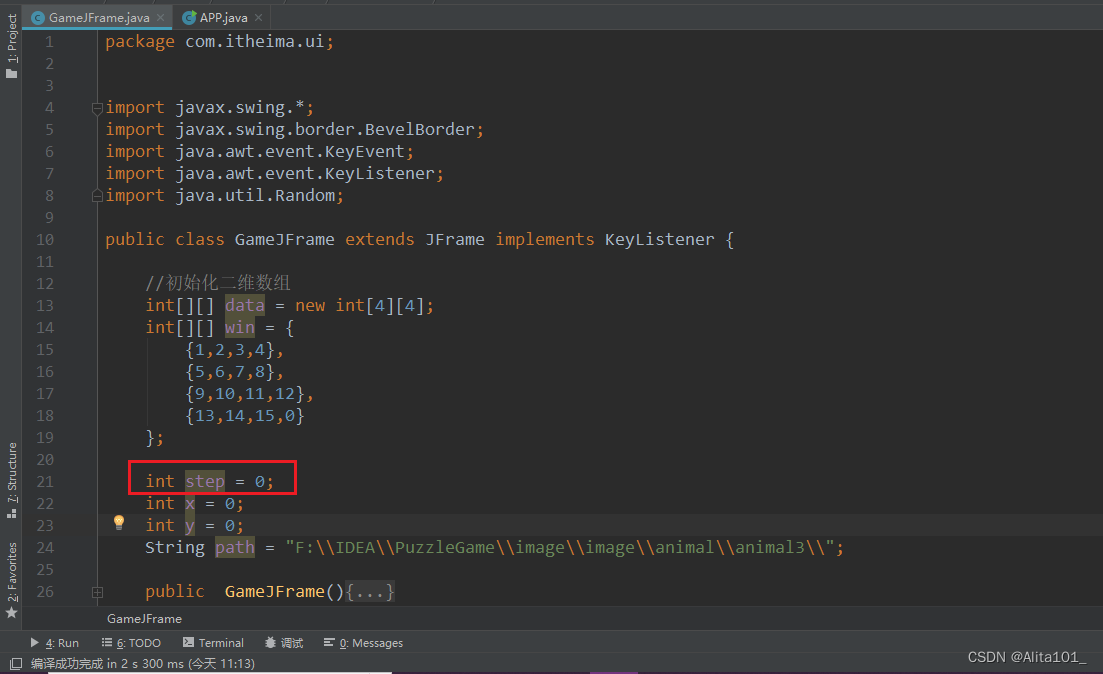# 辗转相除法_欧几里得算法_java的实现（求最大公约数）

+关注继续查看

两个数a，b的最大公约数记为GCD(a,b)。a，b的最大公约数是两个数的公共素因子的乘积。如462可以分解成2 × 3 × 7 × 11；1071可以分解成3 × 3 × 7 × 17。462和1071的最大公约数等于它们共有的素因数的乘积3 × 7 = 21。如果两数没有公共的素因数，那么它们的最大公约数是1，也即这两个数互素，即GCD(a,b)=1。另g=GCD(a,b)，则a=mg, b=ng，其中m，n均为正整数。由上述分析可知，m，n互素。因为m，n没有公共素因子，GCD(m,n)=1。
辗转相除法是一种递归算法。

    private static int gac(int a, int b) {
if(a<b){
swap(a,b);
}
if(b==0)
return a;
else
return gcd(b,a%b);

}

private static void swap(int a, int b) {
a=a^b;
b=a^b;
a=a^b;
}

private static int gac(int a, int b) {
if(a<b){
swap(a,b);
}
while(b!=0){
int c = a%b;
a=b;
b=c;
}
return a;
}

private static void swap(int a, int b) {
a=a^b;
b=a^b;
a=a^b;
}

2个数a,b;已知最大公约数为n；|
8月前
|

Java实现单词计数MapReduce

239 0
|
8月前
|
Java
Java 实现汉字按照首字母分组排序
Java 实现汉字按照首字母分组排序
394 0
|
8月前
|

Java实现图书管理系统

265 0|
8月前
|
Java Windows Spring
java实现spring boot项目启动时，重启Windows进程
java实现spring boot项目启动时，重启Windows进程
420 0
|
8月前
|

Java实现拼图小游戏（1）—— JFrame的认识及界面搭建

333 0|
8月前
|

|
8月前
|

Java实现拼图小游戏（7）—— 计步功能及菜单业务的实现

171 0|
8月前
|
Java
Java实现拼图小游戏（7）—— 作弊码和判断胜利

160 0|
8月前
|
Java
Java实现拼图小游戏（7）——查看完整图片（键盘监听实例2）

127 0